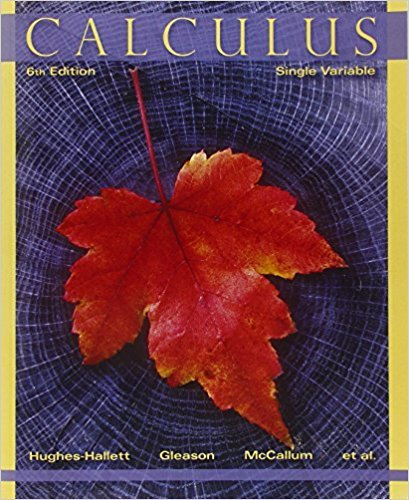×
×

# Solutions for Chapter Chapter 4: USING THE DERIVATIVE## Full solutions for Calculus: Single Variable | 6th Edition

ISBN: 9780470888643Solutions for Chapter Chapter 4: USING THE DERIVATIVE

Solutions for Chapter Chapter 4
4 5 0 280 Reviews
23
0
##### ISBN: 9780470888643

Since 108 problems in chapter Chapter 4: USING THE DERIVATIVE have been answered, more than 32292 students have viewed full step-by-step solutions from this chapter. This expansive textbook survival guide covers the following chapters and their solutions. Calculus: Single Variable was written by and is associated to the ISBN: 9780470888643. Chapter Chapter 4: USING THE DERIVATIVE includes 108 full step-by-step solutions. This textbook survival guide was created for the textbook: Calculus: Single Variable , edition: 6.

Key Calculus Terms and definitions covered in this textbook
• Angle of depression

The acute angle formed by the line of sight (downward) and the horizontal

• Conic section (or conic)

A curve obtained by intersecting a double-napped right circular cone with a plane

• Dot product

The number found when the corresponding components of two vectors are multiplied and then summed

• Five-number summary

The minimum, first quartile, median, third quartile, and maximum of a data set.

• Gaussian elimination

A method of solving a system of n linear equations in n unknowns.

• Graphical model

A visible representation of a numerical or algebraic model.

• Magnitude of a real number

See Absolute value of a real number

• Obtuse triangle

A triangle in which one angle is greater than 90°.

• Partial fractions

The process of expanding a fraction into a sum of fractions. The sum is called the partial fraction decomposition of the original fraction.

• Quotient rule of logarithms

logb a R S b = logb R - logb S, R > 0, S > 0

The measure of an angle in radians, or, for a central angle, the ratio of the length of the intercepted arc tothe radius of the circle.

The distance from a point on a circle (or a sphere) to the center of the circle (or the sphere).

• Real number line

A horizontal line that represents the set of real numbers.

• Reflection through the origin

x, y and (-x,-y) are reflections of each other through the origin.

• Second quartile

See Quartile.

• Solution of a system in two variables

An ordered pair of real numbers that satisfies all of the equations or inequalities in the system

• Summation notation

The series a nk=1ak, where n is a natural number ( or ?) is in summation notation and is read "the sum of ak from k = 1 to n(or infinity).” k is the index of summation, and ak is the kth term of the series

• Terminal side of an angle

See Angle.

• Unit vector

Vector of length 1.

• Venn diagram

A visualization of the relationships among events within a sample space.

×